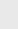Course Content

0/4

0/3

0/1

0/2

0/1

0/4

0/3

0/5

0/3

0/5

0/5

0/1

0/6

0/2

0/4

0/5

17.2 Logarithm 对数

1. Simplify 化简
(a)(b)+(c) log25 + log2log50 + (log2)(a)(b) 0

(c) 2

2.

If a+ b= 14ab, prove that=3.

Given that 2= 9= 125= 120, prove that++=1

4.

Given that a, b, c are positive and 3= 4= 6, prove that=+.